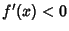## First Derivative TestSupposeis Continuous at a Stationary Point.

1. Ifon an Open Interval extending left fromandon an Open Interval extending right from, thenhas a Relative Maximum (possibly a Global Maximum) at.

2. Ifon an Open Interval extending left fromandon an Open Interval extending right from, thenhas a Relative Minimum (possibly a Global Minimum) at.

3. Ifhas the same sign on an Open Interval extending left fromand on an Open Interval extending right from, thendoes not have a Relative Extremum at.

See also Extremum, Global Maximum, Global Minimum, Inflection Point, Maximum, Minimum, Relative Extremum, Relative Maximum, Relative Minimum, Second Derivative Test, Stationary Point

References

Abramowitz, M. and Stegun, C. A. (Eds.). Handbook of Mathematical Functions with Formulas, Graphs, and Mathematical Tables, 9th printing. New York: Dover, p. 14, 1972.